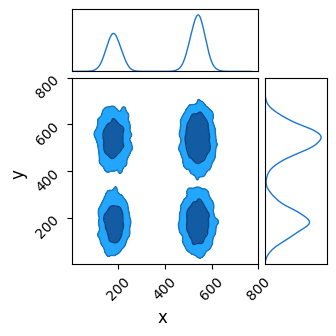# Initialize MCMC Chains from Multiple Predetermined Starting Points

Hello

I’m trying to run an an `MCMC` chain on a multi-modal distribution, and am trying to use `nested sampling` to get a rough first pass place of the warmup. I’m looking for a way to take the `NS` chain, which is has already converged on the different islands of high likelihood, and use these points, or some subset of them, as a starting condition for several `HMC` chains for the main sampling run.

`infer.initialization.init_to_value` only seems to work for a single starting point, not a set of them. I’ve managed awkward solutions like creating a new `infer.MCMC` object for each chain / starting position, but this hardly seems like the best approach.

Hi @Hourglass, currently, we allow to pass in `mcmc.run(..., init_params=init_params)` which works for multiple chains, but those parameters lie in unconstrained domains. You can convert samples from the constrained domain to the unconstrained domain by using something like

``````init_params = jax.vmap(lambda p: unconstrain_fn(model, model_args, model_kwargs, p))(init_values)
``````

The `unconstrain_fn` is not available yet. Please express your interest in Inverse bjiector transformation (from constrained to unconstrained space) · Issue #1554 · pyro-ppl/numpyro · GitHub .Maybe we can add documentation to MCMC class to illustrate this usage?

I’ve tried using `init_params` in `mcmc.run()`, but haven’t had much luck, I must be doing something wrong here.

``````#Generate starting chain positions
nchains = 5
start_positions = ns.get_samples(jax.random.PRNGKey(1), nchains )

#Create MCMC object (use small sample / chain numbers for testing)
sampler = numpyro.infer.MCMC(
infer.NUTS(model = model),
num_warmup=0,
num_samples=3,
num_chains=nchains ,
progress_bar=False,
chain_method = 'sequential',
)

#Run chain using starting positions in start_positions
sampler.run(jax.random.PRNGKey(10), init_params=start_positions)
``````

However, when I run this my chain doesn’t seem to be starting at any of the points in start_positions and doesn’t appear to be properly searching out parameter space.

``````
Starting Positions
x :	 [122.10673 191.90416 206.53766 508.39587 519.9441 ]
y :	 [516.23395 507.63416 499.23264 477.45142 249.01839]
Total No. Samples in main chain:
(15,)
x :	 [460.97742 460.97742 460.97742 247.99597 247.99597 247.99597 192.64108 192.64108 192.64108 436.63904 436.63904 436.63904 483.02737 483.02737 483.02737]
y :	 [228.3003  228.3003  228.3003  657.22626 657.22626 657.22626 699.5137 699.5137 699.5137 95.40972  95.40972  95.40972 135.28629 135.28629 135.28629]
``````

I’ve tried a number of variations, including using a different random seed for each chain and running the chains one at a time then collecting them, but I keep finding myself going in circles. There must be a more elegant way to use ‘n’ points from start_positions as the starting positions for the ‘n’ chains. Have I missed something obvious here?

The only thing I’ve found that works is creating a new infer.mcmc() object for each chain and feeding a different starting point to init_to_value(), but this seems like a clunky way to do things

Ah sorry, could you install the dev branch instead?

``````pip install git+https://github.com/pyro-ppl/numpyro.git
``````

I just ran the same code with the dev branch of NumPyro and the latest version of JAXNS, and now the chains are behaving even more strangely:

``````Starting Positions
x :	 [587.36707 522.5249  156.05652 223.1214  216.75415]
y :	 [482.78903 541.8898  493.17975 521.8364  560.7812 ]
Total No. Samples in main chain:
(15,)
x :	 [7.999999e+02 7.999999e+02 7.999999e+02 7.999999e+02 7.999999e+02 7.999999e+02 7.999999e+02 7.999999e+02 7.999999e+02 9.403955e-36 9.403955e-36 9.403955e-36 7.999999e+02 7.999999e+02 7.999999e+02]
y :	 [7.999999e+02 7.999999e+02 7.999999e+02 7.999999e+02 7.999999e+02 7.999999e+02 7.999999e+02 7.999999e+02 7.999999e+02 9.403955e-36 9.403955e-36 9.403955e-36 7.999999e+02 7.999999e+02 7.999999e+02]
``````

My model draws ‘x’ and ‘y’ from Uniform(0,800), so it seems like this is somehow hugging the boundaries of the parameter space. For reference, my model is:

``````def potential(x,y):
x_width = 30
y_width = 60

out = 0
for i in [0,1]:
for j in [0,1]:
dx = x - (180 + 360*i)
dy = y - (180 + 360*j)
r2 = (dx/x_width)**2 + (dy/y_width)**2
out += jnp.exp(-r2/2)

return(out)

def model():

x = numpyro.sample('x', numpyro.distributions.Uniform(0,800))
y = numpyro.sample('y', numpyro.distributions.Uniform(0,800))

numpyro.factor('log_pot', jnp.log( potential(x,y) + 1E-15 ) ) #Add buffer to avoid log-zero issues which mess with some samplers
``````

Just to double check, I ran the model with pure HMC and init_to_uniform() and it worked finecould you transform your samples into unconstrained domain before using it as `init_params`? see Initialize MCMC Chains from Multiple Predetermined Starting Points - #2 by fehiepsi

To clarify, is the correct way to do this:

``````start_positions = ns.get_samples(jax.random.PRNGKey(1),5)

from numpyro.infer.util import unconstrain_fn
init_params = jax.vmap(lambda p: unconstrain_fn(model, model_args, model_kwargs, p))(start_positions)
...create sampler etc...
sampler.run(jax.random.PRNGKey(10), init_params=init_params)
``````

yes, but unconstrain_fn is not available yet.Ah, so this isn’t doable yet? There’s no way to start with nested sampling for burn-in and then switch the HMC for the sampling?

If that’s the case, what is the easiest way to change the termination condition for the nested sampling run so that it acquires cleaner contours in the latest version of NumPyro? I know JAXNS can adjust the number of points and the termination conditions, but I’ve had issues changing these in my NumPyro models

You need to manually transform your samples to unconstrained space. For example, to perform such transformation for Uniform(0, 8), you can do

``````unconstrained_x = dist.biject_to(dist.Uniform(0, 8).support).inv(x)
``````

You can also use `transform_fn`

``````from numpyro.infer.util import transform_fn

transforms = {"x": dist.biject_to(dist.Uniform(0, 800).support),
"y": dist.biject_to(dist.Uniform(0, 800).support)}
params = transform_fn(transforms, samples, invert=True)
``````

Thank you, this has worked! I’m having issues with the step-sizes of these chains being too large, as there has been no burn-in phase to adapt it, but I’m sure there’s a way I can set that manually.

Hi @fehiepsi, is the `unconstrain_fn` available now?